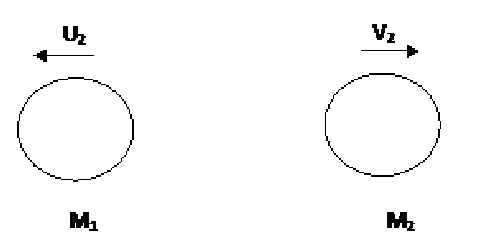Custom Search
If you want to discuss more on this or other issues related to Physics, feel free to leave a message on my Facebook page.

## Tuesday, February 23, 2010

### Conservation of linear momentum in collisions

As we have seen in this previous post, in a close system the sum of linear momentum is a constant. Hence in any collision if the sum of linear momentum of the objects before the collision would be equal to the sum of linear momentum after the collision.

Fig 1 below shows two spherical objects travelling toward each other. The mass of the two objects are 1.0 kg each. Object 1 is travelling at a velocity of 5.0 m/s  and object 2 is travelling at a velocity of –5.0 m/s.Fig 1

If  p1b is the linear momentum of object 1 before the collision and p2b

is the linear momentum of  object 2 before the collision then

the sum of linear momentum = p1b +p2b

= m1u1 + m2v1

=1.0*5.0 + 1.0*(-5.0)

= 5.0 - 5.0

=0 Kg m/s

As you can see before the collision the sum of the linear momentum is 0 kg m/s. According to the principle of conservation of linear momentum the sum of linear momentum at all time will thus be 0 kg m/s before and after the collision.

Fig 2 below shows the spherical objects after the collision while they are separating. According to the principle of conservation of linear momentum after the collision the sum of linear will thus be 0 kg m/s.

Question

If after the collision the two objects separate such that object 2 moves with a velocity of  5.0 m/s, calculate the velocity of object 1.Fig 2

If  p1a is the linear momentum of object 1 after the collision and p2a

is the linear momentum of  object 2 after the collision then

the sum of linear momentum = p1a +p2a

= m1u2 + m2v2

=1.0*u2  + 1.0 *5.0

= u2  +5.0

Now if we use the principle of  of conservation of linear momentum

sum of linear momentum before the collision = sum of linear momentum before the collision

0 =   u2  +5.0

u2  = - 5.0 m/s